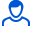Content: teorver_5586.doc (28.00 KB)

Positive responses: 0
Negative responses: 0

Refunds: 05586. x̅=115.52, n=100, σ=10. Find the confidence interval for estimating with a reliability of 0.99 the unknown mathematical expectation a of a normal distributed feature X of the general population, knowing the sample mean x̅=115.52, the sample size n=100 and the standard deviation σ=10.
Detailed solution. Decorated in Microsoft Word 2003 (Quest decided to use the formula editor)
No feedback yet